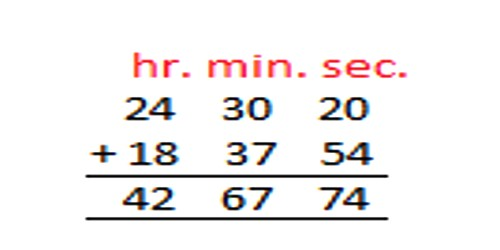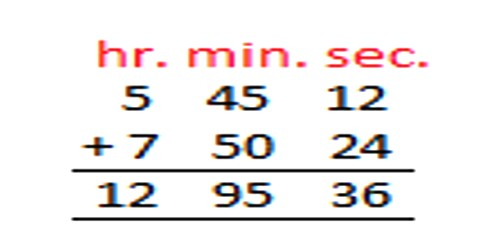Mathematic

# How to Change Hours and Minutes to Seconds?Changing Hours and Minutes to Seconds

Add or Subtract the hours and minutes separately. But you may need to do some adjusting if the minutes end up 60 or more, or less than zero!

How to find the number of minutes if hours and minutes are known:

• Multiply the number of hours by 60.

Addition System: Add 5 hr. 45 min. 12 sec. and 7 hr. 50 min. 24 sec.12 hr. 95 min. 36 sec.

= 12 hr. + (60 min. + 35 min.) + 36 sec.

= 12 hr. 1 hr. + 35 min. + 36 sec.

= 13 hr. 35 min. 36 sec.

Example 1: What is 2:45 + 1:20?

Add the Hours: 2 + 1 = 3

Add the Minutes: 45 + 20 = 65

The minutes are 60 or more, so subtract 60 from minutes (65 − 60 = 5 Minutes)

and add 1 to Hours (3 + 1 = 4 Hours)

Example 2: What is 4:10 – 1:35  ?

Subtract the Hours: 4 − 1 = 3

Subtract the Minutes: 10 − 35 = − 25

The minutes are less than 0, so; add 60 to Minutes (−25 + 60 = 60 − 25 = 35 Minutes) and subtract 1 from Hours (3 − 1 = 2 Hours)